Skip to main content
It looks like you're using Internet Explorer 11 or older. This website works best with modern browsers such as the latest versions of Chrome, Firefox, Safari, and Edge. If you continue with this browser, you may see unexpected results.

# R

## Charts and Graphs in RStudio

Making charts and graphs in RStudio is easy!

Charts and graphs are effective ways to represent data to an audience.

## Select Desired Graph

A pie chart is best for showing the proportions of occurrence of the options in a nominal level variable.

Code

pie(table(SLID$language)) We are graphing a pie chart of the contingency table with the variable language from the SLID dataset. Output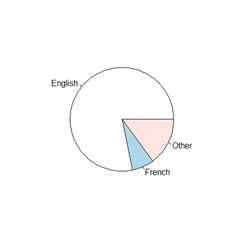The pie chart shows us that the overwhelming majority of observations reported speaking English, the next largest group is other languages, and the smallest French. This a quick and effective way to share the percent of observations compared with others. It iis often that publications do not accept color charts, so using patterns instead are also effective. Histograms are best to plot continuous level variables because, as the name suggests, the values are on a continuum. Histograms are very helpful for investigating the distribution of continuous variables which is important for determining if a variable needs to be recoded. Code hist(SLID$age)

We are graphing a hist (histogram) of the variable age from the dataset SLID.

Output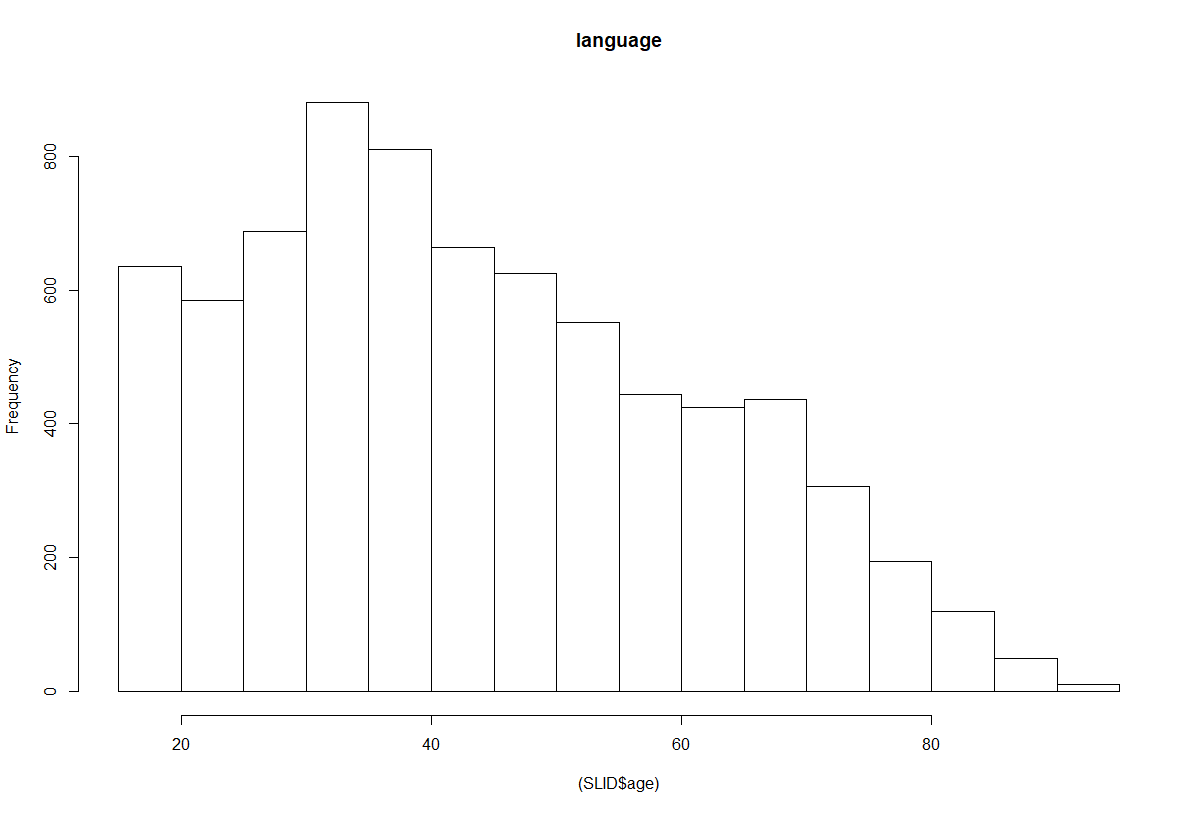The histogram shows us the range of ages among the observations and the frequency of occurrence. We can also see that the distribution of age does not follow a normal curve and is skewed to the right. This may effect our results of our earlier statistical tests. Sas reports the percent of frequencies of the whole dataset, rather than raw counts.

Boxplots, often called box-and-whisker plots and are used to represent the quartiles of continuous level variables. Boxplots display the variation in the sample with boxes that represent the quartiles and 'whiskers' of observations outside the upper and lower quartiles. These plots can be done with a single variable or multiple variables, as we will see below.

Code

boxplot(SLID$age) We are graphing a boxplot of the variable age from the dataset SLID Output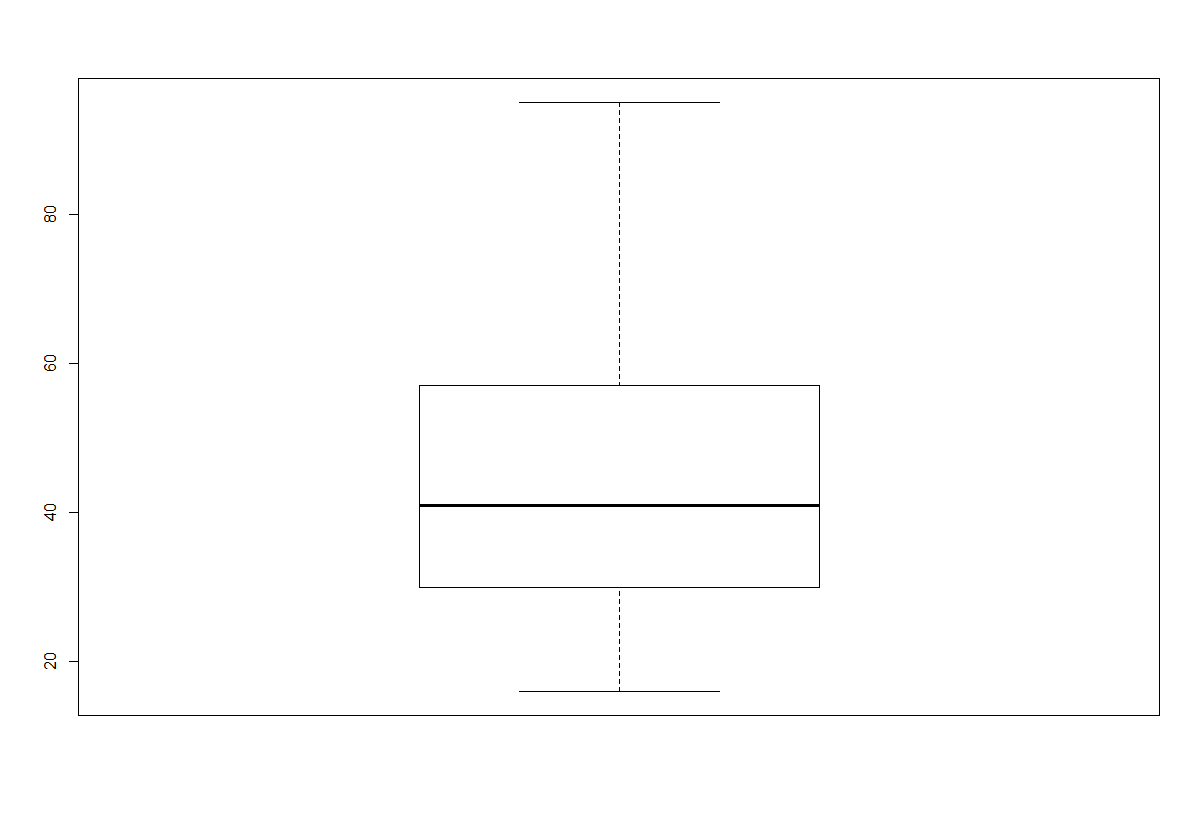The box plot below shows us the median (just above 40) of the variable age with a horizontal line inside the blue box. The top and bottom edges of the blue box are the 25 (Q1) and 75 (Q3) quartiles of the distribution. Next, the whiskers are the minimum and maximum values recorded for age of the observations. Code boxplot(age ~ sex, data=SLID) We are graphing a boxplot of age ~ (by) the variable sex using data from the SLID dataset. Output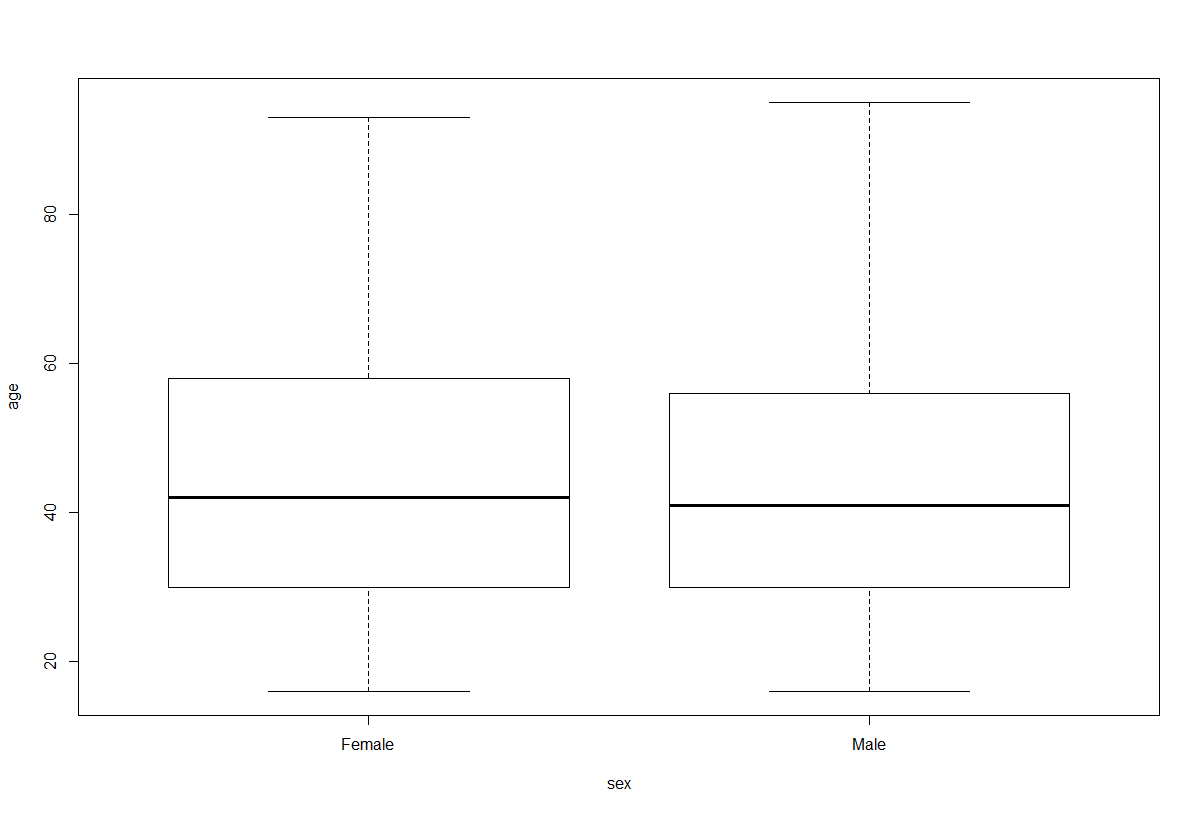This box plot is separated by the sex of the observations (Female and Male). This helps us to see the distribution of age by sex. Bar charts are bested used to represent ordinal level variables to show the distribution of the options. We can graph a bar chart of a single variable or multiple variables for a direct comparison. Code barplot(table(SLID$language), main="language"

We are graphing a barplot of the contingency table with the variable language from the SLID dataset. The main label for the graph is language

Output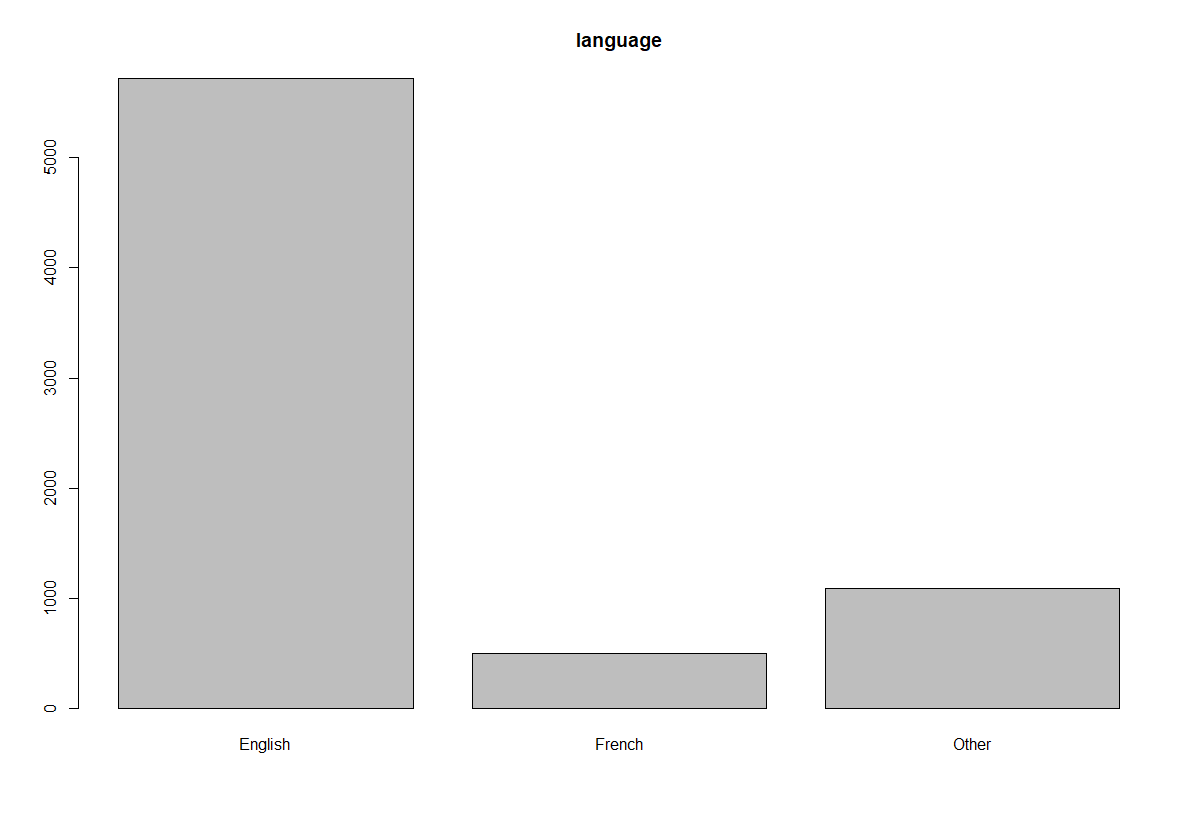The bar chart above shows the raw count of observations of the variable sex broken up by the observations. We can clearly see that there are more females than males in the dataset, but this difference is not great. Using the results from this bar chart we could ask ourselves "Is there a statistically significant difference between females and males across language, education, or wages?".

Code

barplot(table(SLID\$language, SLID\$sex), xlab="sex", ylab="frequency")

We are graphing a barplot of the contingency table with the variables language and sex from the SLID dataset. The x-axis (xlab) is labeled sex and the y-axis (ylab) is labelefrequency

Output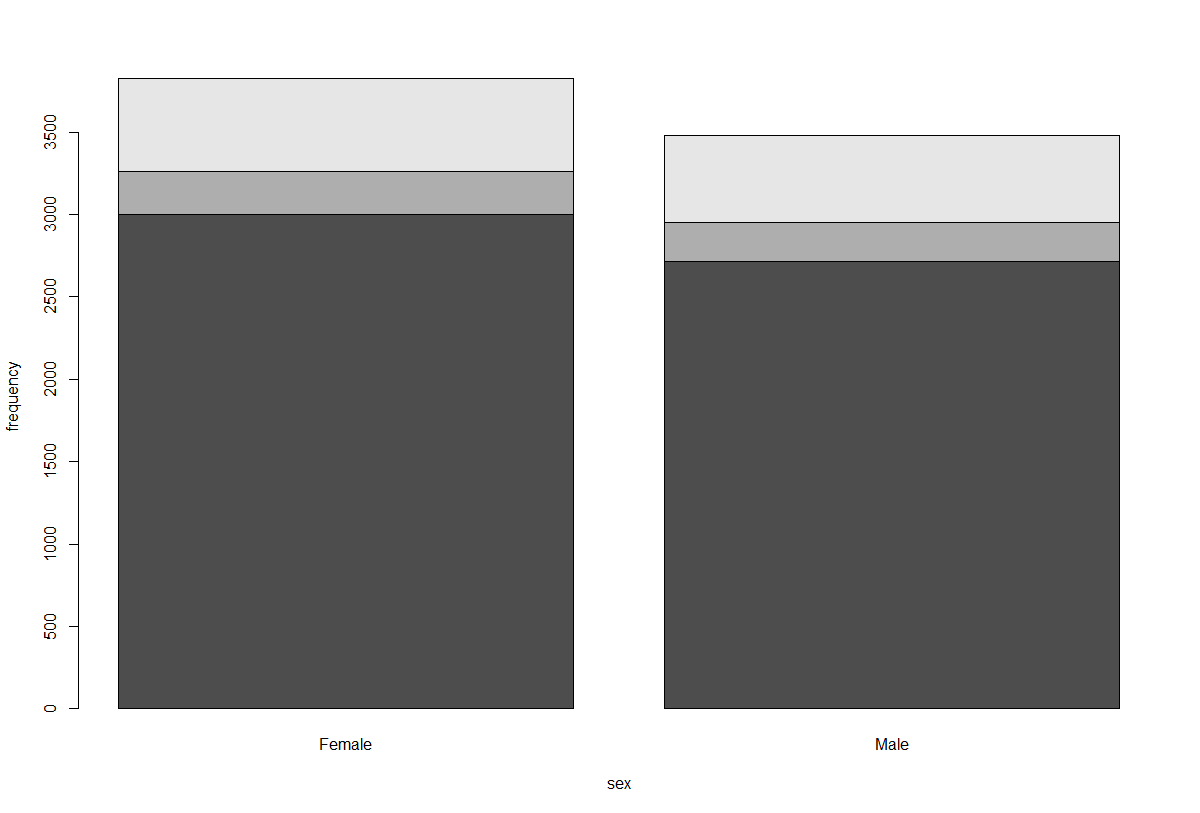We have broken the observations by sex (female and male) and the mean of age within each group.

Scatter plots are best used to graphically show if there is a relationship between two variables and what that relationship may look like.

Code

scatterplot(wages~education, regLine=FALSE, smooth=FALSE, boxplots=FALSE, data=SLID)

We are doing a scatterplot of the variables wages by education. We are not (FALSE) including a regLine (regression line) in the plot, a smooth line, and boxplots. The data for the scatter plot comes from the SLID dataset.

OutputAbove is a scatter plot of the variables education by wages. That is, the points in this graph are the values of education relative to wages. Scatter plots are very helpful when examining continuous level variables and if a graphical relationship exists. We can see in this scatter plot that there is some clustering of observations when educations is 15 and wages is 10. This suggests there may be some relationship that exists. After looking as this graph, we would next want to conduct statistical tests to see if the relationships is statically significant.